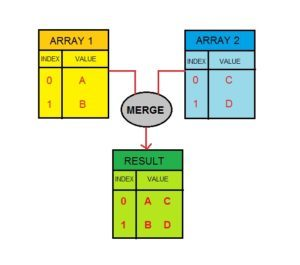# How to merge the first index of an array with the first index of second array?

• Last Updated : 08 Jun, 2020

The task is to merge the first index of an array with the first index of another array. Suppose, an array is array1 = {a, b, c} and another array is array2 = {c, d, e} if we perform the task on these arrays then the output will be

```result array
{
=> array(2)
{
=> string(1) "a"
=> string(1) "c"
}
=> array(2)
{
=> string(1) "b"
=> string(1) "d"
}
=> array(2)
{
=> string(1) "c"
=> string(1) "e"
}
}
```Most people think array_merge() function can resolve the above requirement the following code shows how this isn’t the way to achieve it:

Example 1: Using the array_merge() function will give you the desired result.

## php

 ``

Output:

```array(6) { => string(1) "a"
=> string(1) "b"
=> string(1) "c"
=> string(1) "c"
=> string(1) "d"
=> string(1) "e"
}

```

In order to combine the two arrays by indices, we have to loop through them and merge as we go. Such that the first index of the first and second array together form the first index of the resultant array.

Example 2: Program to merge 2 simple arrays

## php

 ```\$value` `){``      ``\$val``=``\$array2``[``\$key``];``      ``\$result``[``\$key``]=``array``(``\$value``,``\$val``);``    ``}`` ` `    ``var_dump(``\$result``);``?>`

Output:

```array(3) {
=> array(2)
{
=> string(1) "a"
=> string(1) "c"
}
=> array(2)
{
=> string(1) "b"
=> string(1) "d"
}
=> array(2)
{
=> string(1) "c"
=> string(1) "e"
}
}

```

Example 3: Program to merge 2 complex arrays

## php

 ```\$value` `){``      ``\$val``=``\$array2``[``\$key``];``      ``\$result``[``\$key``]=``array``(``\$value``,``\$val``);``    ``}`` ` `    ``var_dump(``\$result``);``?>`

Output:

```array(2) {
=> array(2)
{
=> array(2)
{
=> string(1) "a"
=> string(1) "b"
}
=> array(2)
{
=> string(1) "z"
=> string(1) "y"
}
}
=> array(2)
{
=> array(2)
{
=> string(1) "c"
=> string(1) "d"
}
=> array(2)
{
=> string(1) "x"
=> string(1) "w"
}
}
}

```

My Personal Notes arrow_drop_up